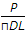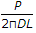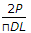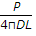# Civil Engineering - UPSC Civil Service Exam Questions

1.

The process of determining the location of the station (on the map) occupied by the plane table is called as

 A. Intersection B. Three-point problem C. Traversing D. Resection

Explanation:

No answer description available for this question. Let us discuss.

2.

Which one of the following correctly expresses the split tensile strength of a circular cylinder of length L and diameter D, subject to a maximum load of P ?

 A.B.C.D.Explanation:

No answer description available for this question. Let us discuss.

3.

If 'A' is the angle formed by two gauge faces, the crossing number will be

 A. tan A B. cot A C. sec A D. A rad

Explanation:

No answer description available for this question. Let us discuss.

4.

What is the curve resistance for a 50 tonnes train on a BG track on a 4° curve?

 A. 0.05 tonne B. 0.06 tonne C. 0.08 tonne D. 0.01 tonne

Explanation:

No answer description available for this question. Let us discuss.

5.

What does high COD to BOD ratio of an organic pollutant represent ?

 A. High biodegradability of the pollutant B. Low biodegradability of the pollutant C. Presence of free oxygen for aerobic decomposition D. Presence of toxic material in the pollutants